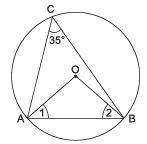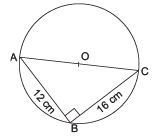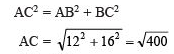Courses

# Very Short Answer Type Questions- Circles Class 9 Notes | EduRev

## Mathematics (Maths) Class 9

Created by: Full Circle

## Class 9 : Very Short Answer Type Questions- Circles Class 9 Notes | EduRev

The document Very Short Answer Type Questions- Circles Class 9 Notes | EduRev is a part of the Class 9 Course Mathematics (Maths) Class 9.
All you need of Class 9 at this link: Class 9

Question 1. In the figure, if ∠ ACB = 35°, then find the measure of ∠ OAB.Solution: ∠AOB = 180° -  (∠1 + ∠2)
= 180° - (35° + 35°)                     [∵ AO = OB ∴ ∠1 = ∠2]
= 110°
∠ACB = (1/2)(∠AOB) = (1/2) (110°) = 55°

Question 2. If points A, B and C are such that AB ⊥ BC and AB = 12 cm, BC = 16 cm. Find the radius of the circle passing through the points A, B and C.
Solution:
∵ AB ⊥ BC
∴ ∠B = 90°                  [Angle in a semicircle]
⇒ AC is a diameterAC = 20

Question 3. The angles subtended by a chord at any two points of a circle are equal. Write true or false for the above statement and justify your answer.
Solution:
False. If two points lie in the same segment only, then the angles will be equal otherwise they are not equal.

Question 4. Two chords of a circle of length 10 cm and 8 cm are at the distance 8.0 cm and 3.5 cm, respectively from the centre, state true and false for the above statement.
Solution
: False. As the larger chord is at smaller distance from the centre.

,

,

,

,

,

,

,

,

,

,

,

,

,

,

,

,

,

,

,

,

,

;